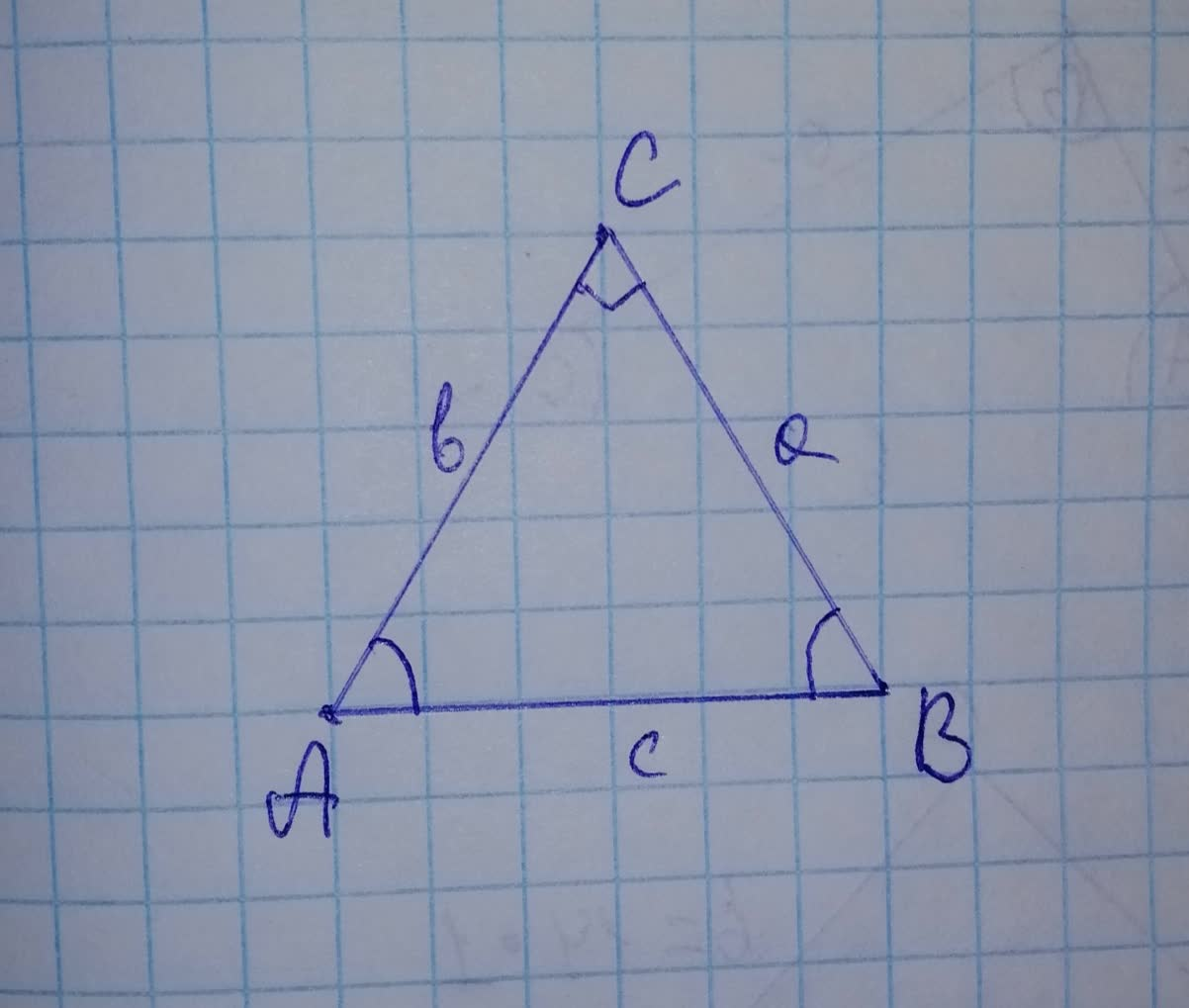# Two sides and an angle are given below. Determine whether the given informationhrostentsp6 2021-11-17 Answered
Two sides and an angle are given below. Determine whether the given information results in one triangles, or no triangle at all. Solve any resulting triangle(s).
$$\displaystyle{b}={4},\ {c}={5},\ {B}={30}^{{\circ}}$$
Select the correct choice below and, if necessary, fill in the answer boxes to complete your choice.
A) A single triangle is produced, where $$\displaystyle{C}\approx?^{{\circ}},\ {A}\approx?^{{\circ}}$$, and $$\displaystyle{a}\approx?$$
B) Two triangles are produced, where the triangle with the smaller angle C has $$\displaystyle{C}_{{{1}}}\approx?^{{\circ}},\ {A}_{{{1}}}\approx?^{{\circ}}$$, and $$\displaystyle{a}_{{{1}}}\approx?$$, and the triangle with the larger angle C has $$\displaystyle{C}_{{{2}}}\approx?^{{\circ}},\ {A}_{{{2}}}\approx?^{{\circ}},$$ and $$\displaystyle{a}_{{{2}}}\approx?$$
C) No triangles are produced.

• Questions are typically answered in as fast as 30 minutes

### Plainmath recommends

• Get a detailed answer even on the hardest topics.
• Ask an expert for a step-by-step guidance to learn to do it yourself.William Yazzie
Step 1
This problem can be solved using Law of sines of triangle. The Law of Sines is the relationship between the sides and angles of non-right triangles.
Simply, it states that the ratio of the length of a side of a triangle to the sine of the angle opposite that side is the same for all sides and angles in a given triangle.for the triangle $$\displaystyle\triangle{A}{B}{C}$$ shown in figure with side lengths a, b, c the law of sines is given as,
$$\displaystyle{\frac{{{a}}}{{{\sin{{A}}}}}}={\frac{{{b}}}{{{\sin{{B}}}}}}={\frac{{{c}}}{{{\sin{{C}}}}}}$$
Step 2
In the question, side $$\displaystyle{b}={4},\ {c}={5},\ {B}={30}^{{\circ}}$$
applying law of sines,
$$\displaystyle{\frac{{{4}}}{{{\sin{{30}}}^{{\circ}}}}}={\frac{{{5}}}{{{\sin{{C}}}}}}$$
$$\displaystyle\Rightarrow{\frac{{{4}}}{{{0.5}}}}={\frac{{{5}}}{{{\sin{{C}}}}}}$$
$$\displaystyle\Rightarrow{8}={\frac{{{5}}}{{{\sin{{C}}}}}}$$
$$\displaystyle\Rightarrow{\sin{{C}}}={\frac{{{5}}}{{{8}}}}={0.625}$$
$$\displaystyle\Rightarrow{C}={{\sin}^{{-{1}}}{\left({0.625}\right)}}={38.68}^{{\circ}}$$
The sum of angles of triangle $$\displaystyle={180}^{{\circ}}$$
therefore
$$\displaystyle\angle{A}={180}-{38.68}-{30}$$
$$\displaystyle\angle{A}={11.32}^{{\circ}}$$
now using Law of sines we can find length of third side.
$$\displaystyle{\frac{{{a}}}{{{\sin{{A}}}}}}={\frac{{{b}}}{{{\sin{{B}}}}}}$$
$$\displaystyle\Rightarrow{\frac{{{a}}}{{{\sin{{111.32}}}}}}={\frac{{{4}}}{{{\sin{{30}}}}}}$$
$$\displaystyle\Rightarrow{\frac{{{a}}}{{{0.931}}}}={\frac{{{4}}}{{{0.5}}}}$$
$$\displaystyle\Rightarrow{a}={\frac{{{4}}}{{{0.5}}}}\times{0.931}={7.45}$$
Step 3
Now let us see if we can have another triangle. We have $$\displaystyle{B}={30}^{{\circ}}$$ given
Now subtract already calculated C from $$\displaystyle{180}^{{\circ}}$$
$$\displaystyle\Rightarrow{C}_{{{2}}}={180}^{{\circ}}-{30}^{{\circ}}-{141.32}^{{\circ}}$$
therefore the third angle will be,
$$\displaystyle{A}_{{{2}}}={180}^{{\circ}}-{30}^{{\circ}}-{141.32}^{{\circ}}$$
$$\displaystyle{A}_{{{2}}}={8.68}^{{\circ}}$$
$$\displaystyle{\frac{{{a}_{{{2}}}}}{{{\sin{{A}}}}}}={\frac{{{b}}}{{{\sin{{B}}}}}}$$
$$\displaystyle\Rightarrow{\frac{{{a}_{{{2}}}}}{{{\sin{{8.68}}}}}}={\frac{{{4}}}{{{\sin{{30}}}}}}$$
$$\displaystyle\Rightarrow{\frac{{{a}_{{{2}}}}}{{{0.15}}}}={\frac{{{4}}}{{{0.5}}}}$$
$$\displaystyle\Rightarrow{a}_{{{2}}}={\frac{{{4}}}{{{0.5}}}}\times{0.15}={1.207}$$
Step 4
Therefore there are two triangles possible for the given measurements $$\displaystyle{b}={4},\ {c}={5},\ {B}={30}^{{\circ}}$$
Triangle 1
$$\displaystyle{C}_{{{1}}}={38.68}^{{\circ}}$$
$$\displaystyle{A}_{{{1}}}={111.32}^{{\circ}}$$
$$\displaystyle{a}_{{{1}}}={7.45}$$
Triangle 2
$$\displaystyle{C}_{{{2}}}={141.32}^{{\circ}}$$
$$\displaystyle{A}_{{{2}}}={8.68}^{{\circ}}$$
$$\displaystyle{a}_{{{2}}}={1.207}$$